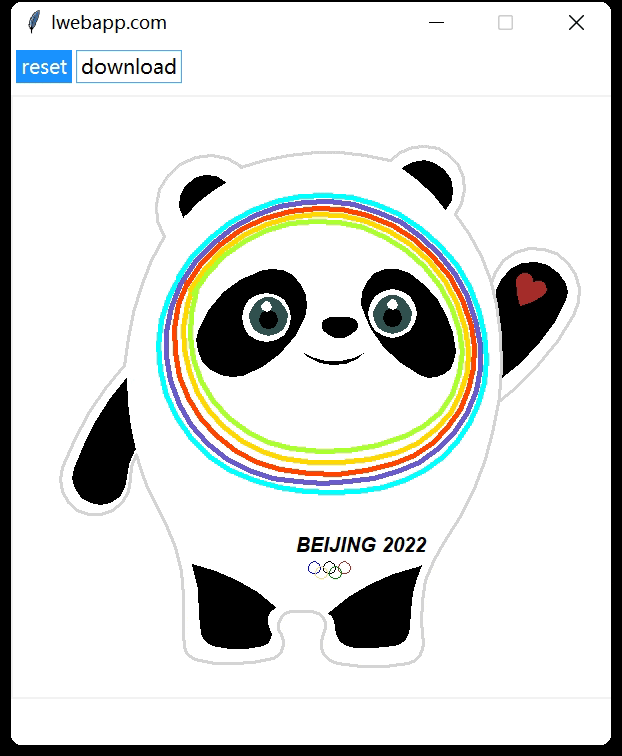# 实现人手一个冰墩墩python画冰墩墩源码

## 背景## 思路和代码

• turtle 用来绘图，有多种绘制方式和样式
• tkinter 用来构建 GUI 程序，也内置了许多组件

``````root = Tk()
root.geometry('600x700+500+60')
root.config(bg='white')
root.title('lwebapp.com')
root.resizable(False, False)
canvas = Canvas(root, width=600, height=600)
canvas.place(x=0,y=50)

t = RawTurtle(canvas)
t.hideturtle()
``````

``````def draw_bdd(t):

# reset
t.penup()
t.home()
t.clear()

t.speed(30)

# left hand
t.goto(177, 112)

t.pencolor("lightgray")

t.pensize(3)

t.fillcolor("white")

t.begin_fill()

t.pendown()

t.circle(-45, 200)

t.circle(-300, 23)

t.end_fill()

``````

``````from PIL import ImageGrab
import os

def save_image(root,widget):
root.update()
x=root.winfo_rootx()+widget.winfo_x()
y=root.winfo_rooty()+widget.winfo_y()

x1=x+widget.winfo_width()
y1=y+widget.winfo_height()
offset = 4
ImageGrab.grab().crop((x + offset,y + offset,x1 - offset,y1 - offset)).save(os.getcwd() + "\\bdd.png", "PNG")
``````

pytxui-draw_bdd

## 总结

• turtle 和 tkinter 结合使用构造 GUI
• 如何构造一个漂亮的 GUI 按钮组件
• 如何将 tkinter Canvas 画布下载为图片